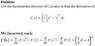# Use fundamental theorem of calculus to compute definite integral

• s3a

## Homework Statement

The problem and my (incorrect) work are typed and attached as TheProblemAndMyWorkTypedUp.jpg.

## Homework Equations

Integral from a to b of f(t) = F(b) – F(a)

## The Attempt at a Solution

As mentioned above, my work is attached as TheProblemAndMyWorkTypedUp.jpg. (The (2 – 1)^12 part “dissappeared” because d/dx (constant) = 0.)

#### Attachments

•TheProblemAndMyWorkTypedUp.jpg
10.7 KB · Views: 451
Replace t with x^2; then t^2 = x^4.

I think you need to change t so that your upper limit is x. Then I think your argument will go through.

Oh, yes, I jumped the gun with assuming it was just x on the upper limit of the integral!

So, this (=the attachment of this reply) is how I do it, right?

Since the upper limit is a function of x, you have to apply chain rule. Straightforward application of FTOC can only be applied when the upper limit is just x and the lower limit a constant.

So, is this (=the attachment of this reply) how it's done?
CorrectYay! Thank you! :)